## 7.1.3 Generating Samples from Probability Distributions

We now turn to a discussion of how to generate sample values (i.e., random observations) of specific random variables. There are at least four different ways of doing this. Throughout this section it will be assumed that we have access to a source of "i.u.d. over [0, 1]" random numbers. We shall denote these numbers by r (or ri, when it is necessary to use an index). Thus, r is a sample value of the random variable R with pdfInversion method. We first consider the most fundamental of the techniques for generating sample values of random variables. It can be applied, at least in principle, in all cases where an explicit expression exists for the cumulative distribution function of the random variable. Basically, the method depends on the fact that, for any random variable Y, the cdf, Fy(y), is a non_ decreasing function with 0 </= Fy(y) </= 1 for all values of y. This suggests a "match" of the cdf with the random numbers, ri. Specifically, by drawing a random number r (see Figure 7.2) and then by finding the inverse image of r on the abscissa through

ys=Fy-1(r) (7.2)a sample value, ys, of random variable Y can be generated. To understand why (see also Figure 7.2) observe that

P{Y</=ys} = P{R</=Fy(ys)} = Fy(ys) (7.3)

the last equality following from the fact that r is uniformly distributed in [0,1]. The following examples illustrate the inversion method.

Example 1: Negative Exponential Random Variable

We have seen that the negative exponential random variable is by far the most common model for the time between urban incidents requiring service. It is therefore essential that we be able to generate random sample values, ts, of the random variable X with the pdf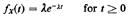As we know, the cumulative distribution of X is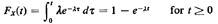Let us then set a random number r1 (uniformly distributed between 0 and 1) equal to Fx(t). We have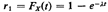or, equivalently,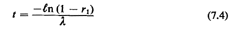Note that (7.4) allows us to draw sample observations of X. That is, each time we wish to draw a sample ts, all we have to do is draw a random number r1 and use (7.4). In fact, since 1 - r1 is also a random number uniformly distributed between 0 and 1, we might as well bypass the subtraction and use directly the random number generated by the computer. Thus, we finally have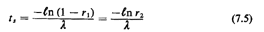where we have used a second random number r2 as a substitute for 1 - r1.

The procedure that we have used is illustrated in Figure 7.3. All we do is draw a random number between 0 and I and then find its "inverse image" on the t-axis by using the cdf. For a numerical example, let us suppose that we have= 2 and that we draw the random number r = 0.168. Then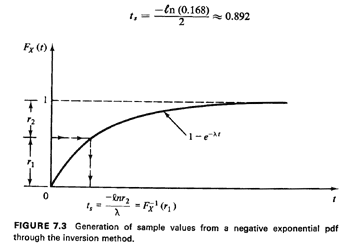Example 2: Locations of Accidents on a Highway

Consider a 6-mile stretch of a highway, lying between the fourth- and tenthmile points of the highway. The spatial incidence of accidents on this 6-mile stretch is shown in Figure 7.4, which indicates that the second half of that stretch is twice as "dangerous" as the first half. We wish to simulate the locations of accidents on the stretch; the locations will be denoted with the random variable X, which is measured from the beginning of the highway at X = 0.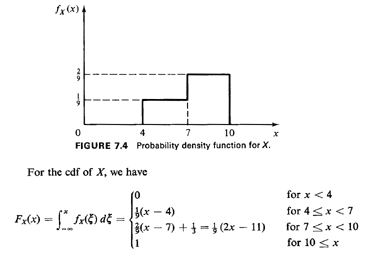This is plotted in Figure 7.5. In the interval of interest, [4, 101, Fx(x) has a breakpoint at x = 7. At that point Fx(x) =1/3. Thus, for values of a random number, r, which are less than 1/3, we should use r = Fx(xs)=1/9(xs- 4) or xs=FX-1 (r) = 9r + 4, while otherwise we should use xs = FX-1(R)= 1/2(9r + 11). This procedure is illustrated in Figure 7.6. Note that the procedure uses a single random number to generate each sample observation, but requires some algebraic work. We can also use an approach that requires no such work if we are willing to generate two random numbers rather than one for each sample observation. This alternative approach is shown by the flowchart of Figure 7.7. Therefore, if the generation of random numbers is not particularly time-consuming (and nowadays it is not), the alternative approach of Figure 7.7 would probably be chosen over the one of Figure 7.6. Many variations, in terms of particular details, of either approach may also be visualized-all leading to a satisfactory generation of random observations of random variable X.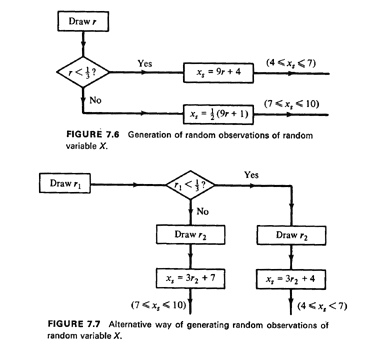In an identical manner, we can use the inversion method to generate amples from discrete random variables. This is shown in Figure 7.8. If {x1, X2, X3, ... 1 are the values that the discrete random variable X can take and px(xk) and Px(xk) are the pmf and cdf, respectively, of X, the method consists of (1) drawing a random number r, and (2) finding the smallest value xi such that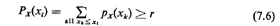In that case we set xs = xi (where xs denotes the sample value of X). Note that entire ranges of values of r now correspond to a single value of X. For instance, if X has a geometric pmf,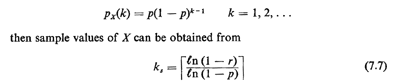where [a] denotes "the smallest integer that is greater than or equal to a."Exercise 7.1 Derive (7.7).

In general, the inversion method is useful in generating sample values of random variables whose cdf's are relatively easy to work with. Many other random variables, whose cdf 's do not fall into this category, can be expressed as functions of these "simple" random variables. Therefore, the inversion method is also important as a "building block" in more sophisticated approaches, such as those described next.

Relationships method. A second approach for generating sample observations of a given random variable is based on taking advantage of some known relationship between this random variable and one or more other random variables. This method has been widely exploited in the case of some of the best-known (and most often used in urban operations research) pmf's and pdf's, such as the Erlang, the binomial, and the Poisson.

Example 3: kth-Order Erlang PDF

We know that the kth-order ErIang random variable X, with pdfcan be considered to be the sum of k independent, identically distributed random variables, each with negative exponential pdf with parameterThat is,Thus, a simple way to generate random observations of X is to generate k random observations from a negative exponential pdf by using (7.5) and then to add these k observations to obtain a single observation of X. That is,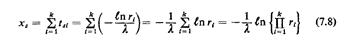The rightmost of these expressions is the most efficient one for computational purposes. 3

Example 4: Binomial PMF

The binomial probability mass function indicates the probability of K successes in n Bernoulli trials. To obtain sample values of K, we simply simulate n Bernoulli trials in the computer. That is, we obtain n random observations of a discrete random variable with probability of "success" and of "failure" equal to p and to 1 - p, respectively, and then count the total number of successes in the n trials. This total number of successes is the sample value of K.

Example 5: Simulating Poisson Events

Consider the Poisson pmf P{N(T) = k), which can be thought of as indicating the probability of observing k events in a time interval T when interarrival times are independent with negative exponential distribution. To generate random observations of N(T) for any given T, we follow the procedure shown on Figure 7.9. We keep generating exponentially distributed time intervals ... [by using (7.5)] until the total length of time represented by the sum of these intervals exceeds T for the first time. That is, we find j such that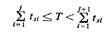Then our sample observation of N(T) is given by ks = j.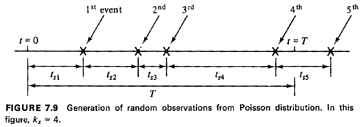Note how in Examples 3-5 the inversion method (for generating sample values of negative exponential or of Bernoulli random variables) is used as a building block (or "subroutine") in the overall procedure.

The rejection method. The rejection method is a quite ingenious approach and is based on a geometrical probability interpretation of pdf's (cf. Section 3.3.2). It can be used for generating sample values for any random variable that:
 1 Assumes values only within a finite range. 2 Has a pdf that is bounded (i.e., does not go to infinity for any value of the random variable).
Let X be such a random variable. Let the maximum value of the pdf fx(x) be denoted as c and let X assume values in the range [a, b] (X need not assume all possible values in [a, b]). To generate random observations of X through the rejection method (see Figure 7.10):STEP 1: Enclose the pdf fx(x) in the smallest rectangle that fully contains it and whose sides are parallel to the x and y axes. This is a (b - a) x c rectangle.4 STEP 2: Using two random numbers, r1 and r2, and scaling each to the appropriate dimension of the rectangle [by multiplying one by (b - a) and the other by c] generate a point that is uniformly distributed over the rectangle. STEP 3: If this point is "below" the pdf, accept the x-coordinate of the point as an appropriate sample value of X. Otherwise, reject and return to Step 2.
The validity of the foregoing procedure is not intuitively obvious on a first reading. We illustrate it first through an example and then explain why it works.

Example 6: Locations of Accidents on a Highway, Revisited

Consider once more the problem of simulating the location of accidents on a 6-mile stretch of highway (Example 2). For the random variable X with pdf fx(x) (Figure 7.4), we have c 2/9, a = 4, and b = 10. Suppose now that the pair of random numbers r1 = 0.34, r2 = 0.81 is drawn in Step 2. These random numbers, with appropriate scaling, identify the point (x1, y1) = [(10 - 4)(0.34) + 4, 2/9(0.81)] = (6.04, 0.18) on the x-y plane. Since at x1 = 6.04, fx(x1) 0. 11 (see Figure 7.4), the point (x1, y1) is found in Step 3 to be above fx(x) and is rejected. No sample value of X has been obtained in this trial. Returning to Step 2, suppose that the new pair of random numbers turns out to be r I = 0.41, r2 = 0. 15. This results in the point (X2, y2) (6.46, 0.033), which is below fx(x). Therefore, the sample value xs = 6.46 is accepted. It is easy to see in this case that the only pairs of random numbers that will be rejected are those for which r1 ‹ 0.5 </= r2.
The reason why this method works is quite simple. The points (x, y) obtained through the procedure of Step 2 are uniformly distributed over the area of the rectangle, (b - a) x c. Therefore, for any point whose x-coordinate is between x0 and x0 + dx (see Figure 7. 10), we have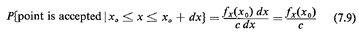Since the x-coordinate of the points (x, y) is chosen randomly in [a, b], the sample observations that will be accepted will then be distributed exactly as fx(x) In other words, were we to obtain a very large number of sample observations, xs, and then construct a histogram of these observations, the histogram would tend to duplicate the shape of fx(x). Note that the rejection method is a very general one since it makes only the two assumptions that we have mentioned regarding the functional form of fx(x). It is especially convenient and easy to use with pdf's which are shaped like polygons. Its main disadvantage is that for some pdf's it may take many rejected pairs of random numbers before an accepted sample value is found.

Exercise 7.2 Show that E[number of trials until an accepted sample value is found] = c(b - a) (7.10)

Note: This is independent of the functional form of fx(x)!

Method of approximations. The fourth approach to generating sample values of random variables consists simply of approximating in some way a probability distribution by another distribution which is simpler to simulate through one of the three earlier methods. The classical example of this type of approach is the generation of random observations from a Gaussian (normal) distribution.

Example 7

Generate random observations of a random variable X with a Gaussian distribution, mean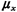and standard deviationSolution

No closed-form expression exists for the cumulative distribution Fx(x) of a Gaussian random variable. Consequently, short of an approximate numerical procedure, we cannot use the inversion method and must resort to some other technique. One approach that can be made arbitrarily accurate and is easy to implement is based on the Central Limit Theorem (CLT): Define a random variable Z as the sum of k independent random variables uniformly distributed between 0 and 1:

Z=R1+R2+R3...+Rk+1+Rk
Now from the CLT we know that for large k, Z has approximately a Gaussian distribution. Since for each Ri we have E[Ri] = 1/2 andthe mean value of Z is k/2 and its standard deviationThus, we can usesince both sides of this equation are equal to a "normalized" Gaussian random variable with mean 0 and standard deviation equal to 1. From (7.11) we finally have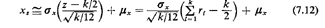where xs is a random observation of random variable X. Generally, values of k greater than 6 or so provide adequate approximations to the Gaussian pdf for values of X within two standard deviations of the mean. The most commonly used value is k = 12, since it simplifies somewhat the computation of (7.12). This means that we have to draw 12 random numbers r1, r2, r12 in order to obtain a single sample value xs of X.
In general, the approximations approach is not always quite as sophisticated as our last example might indicate. Most often, in fact, the approach consists of simply approximating a complicated pdf (or cdf) with, say, a piecewise linear pdf (or cdf) and then using the inversion or the rejection method to generate random observations.

Final comment. Methods for generating samples from some of the standard pdf's and pmf's have been the subject of much research in recent years. Our main purpose above was to present some typical (and correct) approaches rather than the most accurate or efficient method in each example. For instance, excellent alternatives to (7.5) exist for simulating negative exponential pdf's [NEUM 51], [FISH 78].

Similarly, an alternative to (7.12) for the Gaussian random variable with meanand varianceis the following:
 I. Generate two random numbers r1 and r2. 2. Set: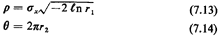3. Obtain samples, xs, of the Gaussian random variable by settingThis method is exact and requires only two random numbers. It can be derived from the analysis described in Example 3 of Chapter 3 (that involved the Rayleigh distribution) and is further developed in Problem 7.2. Many of the more advanced simulation languages/systems (e.g., GPSS, SIMSCRIPT) provide standard internal subroutines for the generation of samples from some of the most common pdf's and pmf's (e.g., the Gaussian, the negative exponential, the Poisson, and the uniform): all that a user of the language/system must do is provide the necessary input parameters. The subroutine then automatically returns a sample value from the specified random variable.

Review example. We review now the first three of the foregoing approaches with an example.

Example 8: Triangular PDF

A hospital is located at the center of a district I- by 1-mile square. Travel is parallel to the sides of the square, and emergency patient demands are distributed uniformly over the square. We wish to generate sample values of the total distance between an emergency patient and the hospital. The pdf for the total distance D = D~ + D, is shown in Figure 7.11.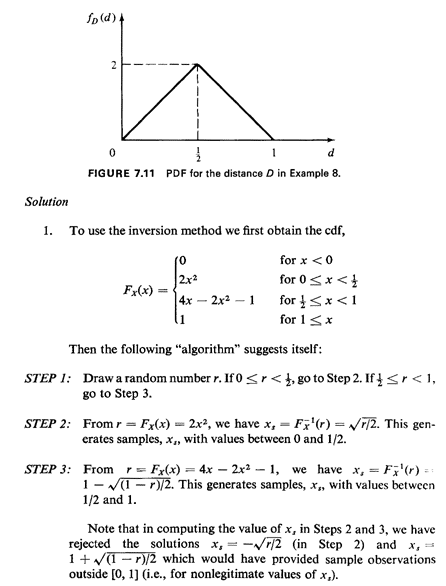2 If we decide to search for a relationship between random variable X and some other random variable(s), we should be able to note immediately that the pdf fx(x) is identical to the one that would have resulted from the definition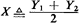where Y1 and Y2 are independent, identically distributed random variables with uniform distributions in [0, 1). (Readers should convince themselves that this is so.) Therefore, a very simple method for generating random observations of X is to usewhere r1 and r2 are the usual random numbers obtained through the random-number generator. This is equivalent to generating samples of the distance traveled in the x-direction, Dx, and in the y-direction, Dy, and adding the two. 3 In using the rejection method, suppose that the first pair of random numbers drawn are r1 = 0.84 and r2 = 0.27. This results in the point (xi, y1) = [1 · (0.84), 2· (0.27)] (0.84, 0.54) in the x-y plane. Since at x1 = 0.84, fx(O.84) = 4(l 0.84) 0.64 (> 0.54), the sample observation is acceptable and we set xs 0.84. Note that in this case 50 percent of the pairs will be accepted on the average. 4 "Most natural": Generate a random point (x = r1, y = r2) in the square and compute3tsi in (7.8) denotes a sample observation of the negative exponential random variable Ti

4 The method will actually work with any rectangle that contains this smallest rectangle. However the probability of rejection will then be greater than with the (b - a) x c rectangle.